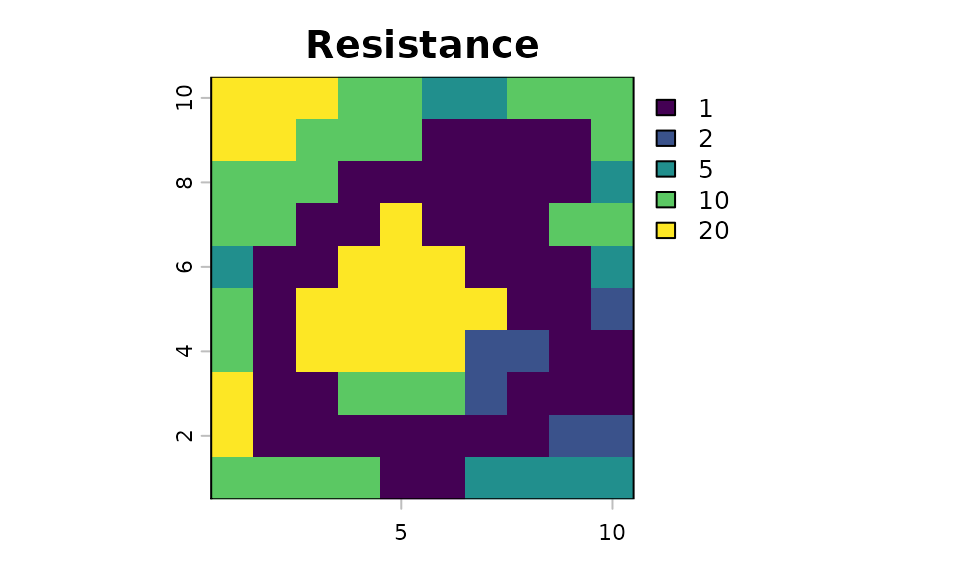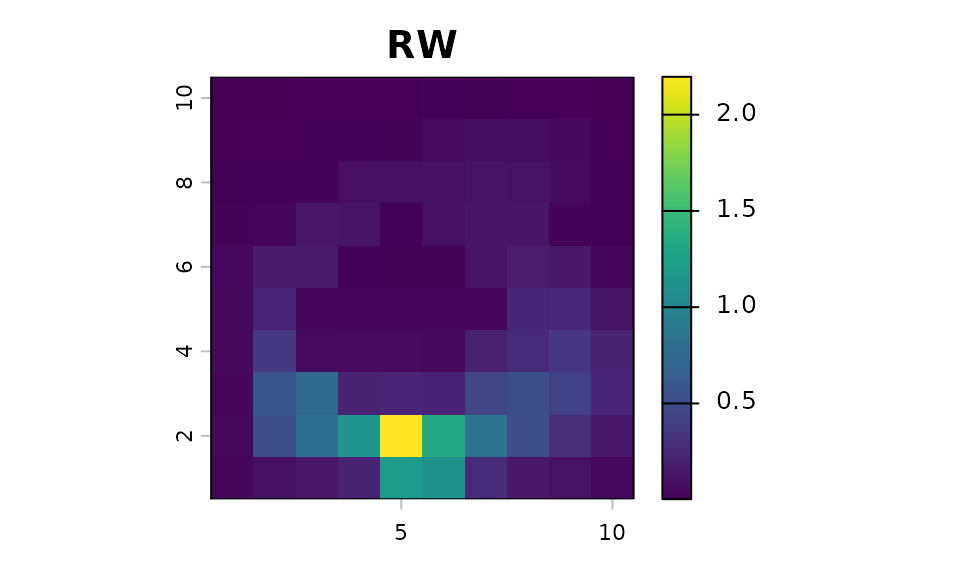## Background

The samc package currently supports two different models: random-walk (RW) and correlated random-walk (CRW). The random-walk is the default model that always has been used by the package. Version 3 made breaking changes to the samc() function to setup a structure for specifying different models, and the correlated random-walk model is the first alternative to make use of this starting with version 3.1.

## Models

Models in the samc package are defined as a list with various components depending on the context:

list(name, fun, dir, sym, dist, kappa)

### Random-Walk

The basic random-walk is the default model used by the package and uses the following properties:

• name: can be omitted or set to "RW"
• fun: a function for calculating transition probabilities from the data input. The convolution algorithm does not allow for custom functions, so this should be omitted in that context.
• dir can be either 4 or 8
• sym can be either TRUE or FALSE, and is used as an optimization when calculating transition probabilities.

### Correlated Random-Walk

The correlated random-walk features are currently experimental. They may not work in all situations and are subject to change.

The correlated random-walk uses the same properties as the RW:

• name: must be set to "CRW"
• fun: a function for calculating transition probabilities from the data input.
• dir can be either 4 or 8
• sym can be either TRUE or FALSE, and is used as an optimization when calculating transition probabilities.

To describe the correlated random-walk behavior, additional parameters are needed to describe the turning behavior:

• dist specifies the name of a distribution or function. Currently, only "vonMises" is supported.
• kappa is specific to the von Mises distribution. It is a single number greater than or equal to 0 that sets a global turning probability.

As the capabilities of the correlated random-walk are expanded in future updates, additional options will be documented here.

To run metrics using the CRW, a single origin and direction value must be supplied in a 1x2 matrix (subject to change). The direction is an integer in the range 1-8 with the directions illustrated as follows:

1 2 3
4   5
6 7 8

For example, 1 is up and left, while 6 is down and left.

Support for multiple origin values, init maps, and dest values is not currently implemented, but will be available future releases once the CRW implementation details are finalized.

## Examples

library("terra")
library("samc")
library("viridisLite")

res_data <- samc::rasterize(example_toy_res)
abs_data <- samc::rasterize(example_toy_res * 0 + 0.05)

plot(res_data, main = "Resistance")

rw_model <- list(fun = "1/mean(x)", dir = 8, sym = TRUE)
crw_model0 <- list(name = "CRW", fun = "1/mean(x)", dir = 8, sym = TRUE, dist = "vonMises", kappa = 0) # Effectively a random walk after the first step
crw_model1 <- list(name = "CRW", fun = "1/mean(x)", dir = 8, sym = TRUE, dist = "vonMises", kappa = 1) # Some bias against turning
crw_model2 <- list(name = "CRW", fun = "1/mean(x)", dir = 8, sym = TRUE, dist = "vonMises", kappa = 2) # Moderate bias against turning
crw_model8 <- list(name = "CRW", fun = "1/mean(x)", dir = 8, sym = TRUE, dist = "vonMises", kappa = 8) # Very strong bias against turning

samc_rw <- samc(res_data, abs_data, model = rw_model)
samc_crw0 <- samc(res_data, abs_data, model = crw_model0)
#> Warning in samc(data, absorption, fidelity, model, options = options): CRW
#> support is currently experimental and may see input changes
samc_crw1 <- samc(res_data, abs_data, model = crw_model1)
#> Warning in samc(data, absorption, fidelity, model, options = options): CRW
#> support is currently experimental and may see input changes
samc_crw2 <- samc(res_data, abs_data, model = crw_model2)
#> Warning in samc(data, absorption, fidelity, model, options = options): CRW
#> support is currently experimental and may see input changes
samc_crw8 <- samc(res_data, abs_data, model = crw_model8)
#> Warning in samc(data, absorption, fidelity, model, options = options): CRW
#> support is currently experimental and may see input changes

origin = 85 # Centered near the bottom
dir = 1 # Up and left

vis_rw <- as.vector(visitation(samc_rw, origin = origin))
vis_crw0 <- as.vector(visitation(samc_crw0, origin = matrix(c(origin, dir), 1)))
vis_crw1 <- as.vector(visitation(samc_crw1, origin = matrix(c(origin, dir), 1)))
vis_crw2 <- as.vector(visitation(samc_crw2, origin = matrix(c(origin, dir), 1)))
vis_crw8 <- as.vector(visitation(samc_crw8, origin = matrix(c(origin, dir), 1)))

plot(map(samc_rw, vis_rw), col = viridis(1024), main = "RW")
plot(map(samc_crw0, vis_crw0), col = viridis(1024), main = "CRW (kappa=0)")
plot(map(samc_crw1, vis_crw1), col = viridis(1024), main = "CRW (kappa=1)")
plot(map(samc_crw2, vis_crw2), col = viridis(1024), main = "CRW (kappa=2)")
plot(map(samc_crw8, vis_crw8), col = viridis(1024), main = "CRW (kappa=8)")